Оценочные платы \$199.00# Обзор

## Ресурсы проектирования

### Design & Integration Files

• Schematic
• Bill of Materials
• Gerber Files
• Allegro Files
• Assembly Drawing Загрузить файлы проектов 1.61 M

### Оценочные платы

Буква "Z" в наименовании компонента указывает на соответствие требованиям RoHS. Отмеченные платы нужны для оценки данной схемы

• EVAL-CN0345-SDZ (\$199.00) Low Power, Multichannel Data Acquisition System with PGIA for Industrial-Level Signals
• EVAL-SDP-CB1Z (\$99.00) Eval Control Board

## Особенности и преимущества

• 18-Bit 8-Channel Data Acquisition System
• Single-ended and differential industrial signal levels
• 750 kSPS per channel switching rate
• 240mW at maximum sampling rate

# Circuit Function & Benefits

The circuit shown in Figure 1 is a cost effective, low power, multichannel data acquisition system that is compatible with standard industrial signal levels. The components are specifically selected to optimize settling time between samples, providing 18-bit performance at channel switching rates up to approximately 750 kHz.Figure 1. Multichannel Data Acquisition Simplified Circuit (All Connections and Decoupling Not Shown)

The circuit can process eight gain-independent channels and is compatible with both single-ended and differential input signals.

The analog front end includes a multiplexer, programmable gain instrumentation amplifier (PGIA); precision analog-to-digital converter (ADC) driver for performing the single-ended to differential conversion; and an 18-bit, 1 MSPS PulSAR® ADC for sampling the signal on the active channel. Gain configurations of 0.4, 0.8, 1.6, and 3.2 are available.

The maximum sample rate of the system is 1 MSPS. The channel switching logic is synchronous to the ADC conversions, and the maximum channel switching rate is 1 MHz. A single channel can be sampled at up to 1 MSPS with 18-bit resolution. Channel switching rates up to 750 kHz also provide 18-bit performance. The system also features low power consumption, consuming only 240 mW at the maximum ADC throughput rate of 1 MSPS.

# Описание схемы

The circuit shown in Figure 1 is a multichannel data acquisition signal chain consisting of a multiplexer, programmable gain stage, ADC driver, and a fully-differential PulSAR ADC. The channel switching and gain switching is synchronized to the conversion period of the ADC.

The system can monitor up to eight channels using a single ADC, reducing component count and cost compared to systems with one ADC per channel. Each channel can be configured with a different gain, allowing for flexibility of input ranges. The effective sample rate for each channel is equal to the sample rate of the ADC divided by the total number of channels being sampled.

The maximum sample rate of the system is limited by the settling time of the components in the analog front end. Multiplexed signals are discontinuous in nature, resulting in potentially large voltage steps between sampling intervals. The components in the signal chain must be given adequate time to settle to these steps before the ADC performs a conversion. To maximize the time given for the signal to settle, the multiplexer channels are switched immediately after the ADC begins a new conversion.

Component Selection

The ADG1207 is a low-capacitance, fast-settling multiplexer that routes one of eight differential inputs to a common differential output. A switching network at the inputs of the ADG1207 adds compatibility with both single-ended and differential input signals. The active channel is selected via the address pins of the device, which are controlled by the SDP-B controller board.

The AD8251 is a programmable gain instrumentation amplifier, that provides selectable gain settings of 1, 2, 4, and 8. The higher gain settings boost smaller input signals to the full-scale input range of the AD7982. Each gain setting has its own suitable input range, which is shown in Table 1.

Table 1. Input Range for Each of the Four Gain Configurations
 Gain Full-Scale Input Range 0.4 ±10.24 V 0.8 ±5.12 V 1.6 ±2.56 V 3.2 ±1.28 V

The AD8475 funnel amplifier provides high-precision attenuation (0.4×), accurate common-mode level shifting, and single-ended to differential conversion. Its low output noise spectral density (10 nV/√Hz) and fast settling time (50 ns to 0.001% for a 2 V output step) make it well suited to drive the AD7982.

The AD7982 is a fully-differential, 1 MSPS, 18-bit PulSAR ADC that features a typical SNR of 96 dB when using a 4.096 V reference. The AD7982 is also low power, and only consumes approximately 7 mW at full throughput. Its power consumption scales with throughput, and can operate at lower sample rates to cut its power use (for example, 70 μW at 10 kSPS).

System DC Accuracy Errors

Figure 2 shows the ideal transfer function of the data acquisition system.Figure 2. ADC Ideal Transfer Function

Each of the components in the data acquisition signal chain have errors that cause the real transfer function of the system to deviate from that in Figure 2. The cumulative effects of these errors can be measured at a system level by comparing dc inputs at the input to the ADG1207 and the resulting output codes from the AD7982. The errors of interest in this system are offset error and gain error.

Offset Error Measurement

For ideal bipolar, differential ADCs, a 0 V differential input results in an output code of 0. Real ADCs typically exhibit some offset error (εb), which is defined as the deviation between the ideal output code and the measured output code for a 0 V input.

The offset error for the data acquisition system can be found by grounding its input and observing the resulting output code. This error varies between each of the gain settings of the AD8251 and between each of the channels of the ADG1207. Offset error is therefore measured for each of the channels in all four gain configurations.

Since the system monitors multiple channels, it is also important to quantify the amount by which the offset error deviates between channels. Offset error match (Δεb, MAX) is a measure of the maximum deviation between the offset error of each of the channels and the average offset error of all of the channels. Offset error match is calculated using the following equation:where εb,i and εb,j are the offset errors for the i and j channels, respectively.

This offset error match can be found for each of the gain configurations. Note that offset error can be expressed either in codes or volts.

Gain Error Measurement

Error in the gain of the system also contributes to overall system inaccuracy. The ideal transfer function of the AD7982 is shown in Figure 2, where the −217 and 217 − 1 output codes correspond to a negative full-scale input voltage (−FS) and a positive full-scale input voltage (+FS), respectively; however, the combination of offset error (εb) and gain error (εm) results in a deviation from this relationship.

Gain error can be expressed as a percentage error between the actual system gain and the ideal system gain. The more common expression is in percent-full-scale error (%FS), which is a measure of the error between the ideal and actual input voltages that produces the 217 − 1 code.

The ideal full-scale input voltage (VFS, IDEAL) is a function of the resolution of the ADC (18-bits for the AD7982) and the accuracy of the reference voltage (VREF). Errors in the voltage reference translate to gain errors in the ADC. To decouple reference errors from ADC gain error, VREF is measured using a precision multimeter. The ideal full-scale input voltage can then be calculated usingThe actual system gain can be found by calculating the slope of the linear regression of a group of several input voltages (mLR) and the resulting output codes:The real full-scale input voltage (VFS, REAL) can then be calculated using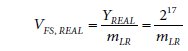The gain error (expressed in %FS error) can then be calculated using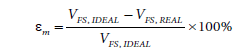The gain error of the system varies with the gain of the AD8251, but is channel independent. Therefore, gain error is measured for each of the four gain configurations, but only using one of the ADG1207 channels in this system.

System Noise Analysis

One of the key design goals in precision data acquisition systems is achieving a high signal-to-noise ratio (SNR), which can be achieved by increasing the full-scale signal amplitude and/or decreasing the noise power generated by the components in the system.

The total noise power present in the system can be found by taking the root sum square (rss) of the noise power contributed by its individual components, referred to the input of the AD7982.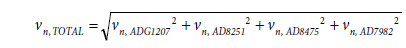The expected SNR of the system (SNREXPECTED) can then be found usingThe expected noise contributions for each component in the system and the resultant expected SNR performance of the whole system is shown in Table 2. The total system noise calculation ignores thermal noise contributed by the passive components in the system.

The noise of the AD7982 ADC is a function of both its inherent quantization error and noise caused by internal components (such as passive components producing thermal noise).

The rms input voltage noise of the AD7982 can be calculated from its specified SNR usingThe SNR for the AD7982 (SNRAD7982) is specified as approximately 96 dB for a 4.096 V reference.

The single-pole RC filter at the input of the AD7982 limits the wide-band noise from the upstream components. A smaller filter bandwidth improves SNR by further limiting noise power; however, its time constant must also be sufficiently short to settle voltage kickbacks due to charge injections that occur as the AD7982 inputs reconnect to the front-end circuitry during the acquisition phase. The appropriate bandwidth for the system is at least 5 MHz (see the Analog Dialogue article, Front-End Amplifier and RC Filter Design for a Precision SAR Analog-to-DigitalConverter for more information).

Noise Due to the AD8475 Funnel Amplifier

The rms noise contributed by the AD8475 (vn, AD8475) is a function of its referred-to-output NSD (eAD8475) and the RC filter bandwidth at the input to the AD7982 (BWRC):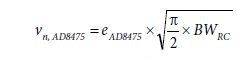Noise Due to the AD8251 Instrumentation Amplifier

The AD8251 functions as a gain stage which improves SNR for small-amplitude signals by boosting their amplitude to more closely fill the ±VREF range at the input to the AD7982. Ideally, if the system gain increases by a factor of G, the SNR (in dB) of the input signal improves by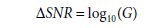This level of improvement is not achievable in reality, however, because wideband noise is also amplified by the noise gain of the circuit. Fortunately, this degradation is not as large as the improvement due to signal gain.

The rms noise contributed by the AD8251 is a function of its referred-to-input NSD (eAD8251), its gain setting (GAD8251), the attenuation factor of the AD8475 (GAD8475), and the noise filter bandwidth at the input of the AD7982:The value of eAD8251 is also dependent on the AD8251 gain and can be found in the AD8251 data sheet.

Noise Due to the ADG1207 Multiplexer

The NSD and resulting rms noise contributed by the ADG1207 can be found using the Johnson/Nyquist noise equation, because the device acts like a series resistance between the source and the rest of the analog front end:andThe resistance of each channel (RON) can be found in the ADG1207 data sheet.

A summary of the calculated noise performance of the system is shown in Table 2. The largest contributors to the total noise are the AD8251 in-amp and the AD7982 ADC.

Table 2. Noise Performance for the Multichannel Data Acquisition System

Settling Time Analysis

When the circuit shown in Figure 1 is sampling multiple channels, each of the different inputs are merged into a time-division multiplexed signal by the ADG1207. Multiplexed signals are discontinuous in nature, and typically have large voltage steps occurring in short time intervals. For the system in Figure 1, the voltage differential between two consecutive channels may be as large as 20 V at the inputs of the ADG1207, and the time allotted for settling is only as long as the sampling period.

Figure 3 shows the settling time model of the circuit in Figure 1. Each of the components in the system has its own settling characteristics (see the following sections).Figure 3. Settling Time Model of CN-0345 Circuit

Settling time is defined as the time required for the analog front-end circuitry to settle an input step to a certain precision. This precision is usually specified in percent error (for example, 0.1% or 0.01%), but in conversion systems it is also helpful to relate it to resolution. For example, settling to a 16-bit resolution is roughly equivalent to settling to 0.001%. Table 3 shows the relationship between settling to percent error and to resolution for a single-pole system.

Table 3. Percent Error and Effective Resolution
 Resolution, No. of Bits LSB (%FS) No. of Time Constants = -In (%Error/100) 6 1.563 4.16 8 0.391 5.55 10 0.0977 6.93 12 0.0244 8.32 14 0.0061 9.70 16 0.00153 11.09 18 0.00038 12.48 20 0.000095 13.86 22 0.000024 15.25

Estimating the settling time of an analog front end with multiple components is not trivial for a variety of reasons. First, many devices do not specify settling characteristics to very high precision. Settling time for an active device is also not linearly related to settling precision, and it may take up to 30 times as long to settle to 0.01% as to 0.1%. This can be due to long-term thermal effects inside the amplifier. Settling time is also dependent on the load that the device is driving, and settling time is generally not characterized for multiple load conditions.

Measuring high-precision settling is also difficult without a specialized characterization platform, due to the effects of oscilloscope overdrive and sensitivity, and the difficulty of generating an input pulse with sufficient rise time and settling time.

Settling time can be estimated provided certain bounds and assumptions are used in analyzing the circuit. The total settling time can be calculated by taking the root sum square (rss) of the settling times of the individual components: Measuring high-precision settling is also difficult without a specialized characterization platform, due to the effects of oscilloscope overdrive and sensitivity, and the difficulty of generating an input pulse with sufficient rise time and settling time. Settling time can be estimated provided certain bounds and assumptions are used in analyzing the circuit. The total settling time can be calculated by taking the root sum square (rss) of the settling times of the individual components:The maximum throughput of the system is inversely proportional to the total settling time:The equivalent circuit for a CMOS switch can be approximated as an ideal switch in series with a resistor (RON) and in parallel with two capacitors (CS, CD). The multiplexer stage and associated filters can therefore be modeled as shown in Figure 4.Figure 4. Settling Time Model of the ADG1207

Each channel functions similarly to an RC circuit having an associated time constant that dominates settling time. Dynamically switching channels complicates signal settling; at the time channels are switched, the difference between the previous output and the current input produces a kickback transient. This kickback is similar to the one that occurs at the input to the AD7982 as it enters the acquisition phase. For a more detailed description, see the Analog Dialogue article, Front-End Amplifier and RC Filter Design for a Precision SAR Analog-to-Digital Converter.

The circuit in Figure 4 was simulated using NI Multisim™, as shown in Figure 5, with the following component values from the respective device data sheets:

• RON = 120 Ω
• CS = 2 pF
• CD = 10 pF
• RIN||CIN = 1.25 GΩ||2 pF

The input resistance of the AD8251 (RIN) is sufficiently large (1.25 GΩ) to be omitted from simulation.Figure 5. Multisim™ Settling Time Model of the ADG1207

The simulation results are shown in Figure 6. The time required for the output of the ADG1207 to settle to 0.001% of 10 V is tS_ADG1207 = 12 ns.Figure 6. Settling Time Waveforms for the ADG1207 Simulation Model

The AD8251 data sheet specifies its settling time for a variety of input voltage step sizes down to a 0.001% error for each gain configuration. Given a load of 10 kΩ and gain setting of 1, the AD8251 can settle a 20 V step at its output to 0.001% in approximately 1 μs. The gain of 1 setting requires the most settling time, so the settling time analysis will use 1 μs.

However, the 1 μs number may not be accurate when the AD8251 is driving one of the inputs of the AD8475, which has an input impedance of 2.92 kΩ instead of 10 kΩ. It is also not possible to ascertain settling time of the AD8251 to 18-bit resolution, due to the nonlinear relationship between settling time and precision. Therefore, the best settling time estimation is 0.001% error (or 16-bit resolution).

The AD8475 has a settling time specification of 50 ns to 0.001% for a 2 V differential output step. The maximum voltage step size expected on the outputs of the AD8475 is twice the reference voltage (VREF), or approximately 8 V. Assuming that the settling time is proportional to the output voltage step, the settling time to 0.001% (16-bits) for an 8 V step will be approximately 200 ns (4 × 50 ns).

The settling time of each amplifier is, therefore,

Settling Time of the RC Noise Filter and AD7982

Figure 7 shows the equivalent circuit of the inputs of the AD7982. REXT and CEXT are the components in the RC wideband noise filter in front of the ADC. RIN and CIN are the input resistance and capacitance of the AD7982, respectively. CIN is mainly the internal capacitive digital-to-analog converter (DAC). CPIN is primarily the pin capacitance, and is ignored. The values for these components are as follows:

• REXT = 10 Ω
• CEXT = 1200 pF
• RIN = 400 Ω
• CIN = 30 pF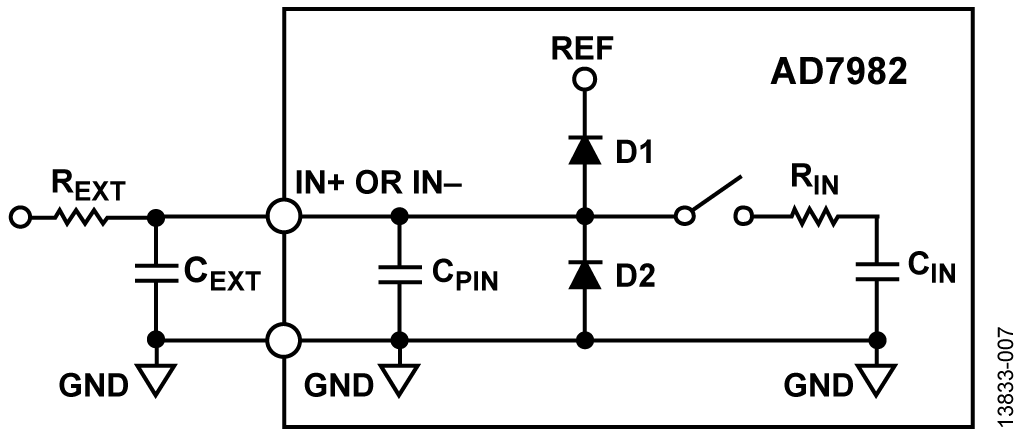Figure 7. Settling Time Model of the AD7982 and RC Noise Filter

The AD7982 employs an internal capacitive DAC and a charge redistribution algorithm to determine its output code. The conversion process contains two phases, acquisition and conversion. During acquisition, the capacitive DAC is connected to the input terminals of the AD7982. During conversion, it is disconnected from the input terminals, and internal logic performs the charge-redistribution algorithm. The maximum specified time of the conversion phase is 710 ns.

The signal must be settled by the end of the acquisition phase for an accurate conversion. In order to maximize the time given for the signal to settle, the multiplexer switches channels immediately after the AD7982 begins its conversion phase.

In addition to settling from the multiplexed signal from the output of the AD8475, the RC noise filter and AD7982 inputs also have to settle to the voltage kickback that occurs at the beginning of the acquisition phase. See the Analog Dialogue article, Front-End Amplifier and RC Filter Design for a Precision SAR Analog-to-Digital Converter.

The settling time for the circuit in Figure 7 was simulated in NI Multisim™ as shown in Figure 8. V1 represents the maximum voltage step expected at either input of the AD7982 (from a single-ended output of the AD8475). CNV and S1 simulate the AD7982 switching from the conversion phase (occurring when V1 changes value) to the acquisition phase (710 ns after start of conversion). CNV keeps S1 open until 710 ns after V1 steps from 0 V to 4 V to represent the transition from the conversion phase to the acquisition phase. ADC_IN is the voltage that is sampled by the AD7982 on a CNV rising edge.

The settling time for this portion of the system is equal to the time between V1 switching to 4 V (at TIME = 0) to ADC_IN settling to 0.001% of 4 V.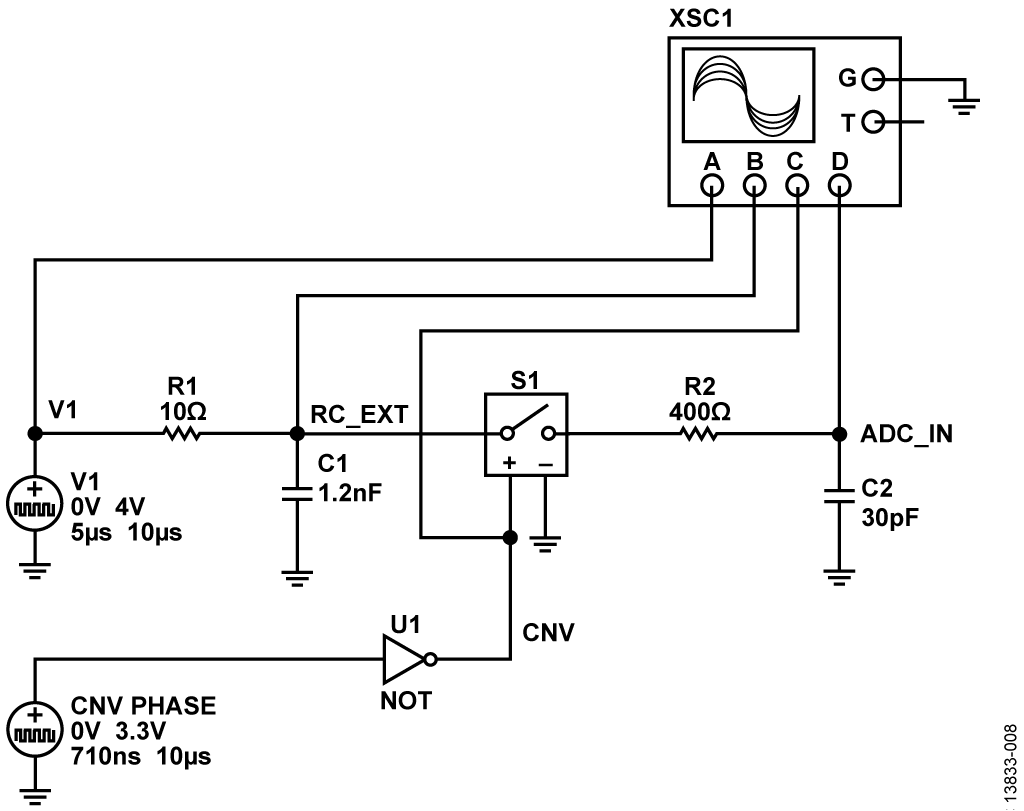Figure 8. Multisim™ Settling Time Model of the AD7982 and RC Noise Filter

The simulation results are shown in Figure 9. The time taken for the output to settle to 0.001% of 4 V is tS_AD7982 = 810 ns.Figure 9. Settling Time Waveforms for the AD7982 and RC Noise Filter Simulation Model

Total System Settling Time

The total settling time of the entire circuit shown in Figure 1 can now be estimated by calculating the rss of the settling times for each component:The expected maximum sample rate of the system is thenOffset and Gain Error Results

Table 4 shows the offset error measured (in LSBs) for each of the channels in each gain configuration for the circuit in Figure 1. Table 4 also shows the average offset error of all of the channels for each gain configuration.

The offset errors were measured by grounding all of the channel inputs and collecting and averaging 32,768 samples taken on each of the channels in each gain configuration.

Table 4. Offset Error Measurements for all Channels and Gain Configurations (Error in LSBs)
 Gain Channel 0 Channel 1 Channel 2 Channel 3 Channel 4 Channel 5 Channel 6 Channel 7 Channel Average Offset Error Match 0.4 −2.34 −2,31 -2,32 −2.29 −2.31 −2.29 −2.28 −2.26 −2.30 0.47 0.8 −2.40 −2,31 −2.33 −2.31 −2.27 −2.26 −2.25 −2.24 −2.30 0.19 1.6 −1.49 −1.34 −1.35 −1.28 −1.31 −1.22 −1.22 −1.14 −1.29 0.47 3.2 0.11 0.34 0.33 0.44 0.47 0.57 0.63 0.73 0.45 0.36

Table 5 shows the gain error measured for each of the gain configurations for the circuit in Figure 1. The %FS error was found using the analysis methods described above, and the actual gain in V/V was calculated by subtracting this error from the ideal gain.

Table 5. Gain Error Measurements for all Gain Configurations
 Gain Gain Error (%FS) 0.4 0.07 0.8 0.05 1.6 0.04 3.2 0.02

Performance Results without Channel Switching

Figure 10, Figure 11, Figure 12, and Figure 13 show the FFT plots for a 10 kHz full-scale sine wave input on a single channel for gain configurations of 0.4, 0.8, 1.6, and 3.2, respectively. Table 6 shows the SNR and rms noise measured for each of the gain configurations.

Table 6. SNR, Noise, and THD vs. Gain for 10 kHz Input
 Gain SNR (dB) RMS Noise (μV rms) THD (dB) 0.4 91.50 77.1 −93.89 0.8 90.36 87.9 −93.97 1.6 89.57 96.2 −93.73 3.2 87.35 124.2 −92.93

The input signal was supplied by an Audio Precision SYS-2700 series signal generator, with the board set in differential input mode. Figure 14 shows total harmonic distortion (THD) measurements vs. the frequency of the input signal for each gain configuration. These results match the THD typical performance characteristic plot from the AD8251 data sheet.Figure 10. FFT for 10 kHz, 20 V p-p Input for Gain = 0.4 on Single, Static ChannelFigure 11. FFT for 10 kHz, 10 V p-p Input for Gain = 0.8 on Single, Static ChannelFigure 12. FFT for 10 kHz, 5 V p-p Input for Gain = 1.6 on Single, Static Channel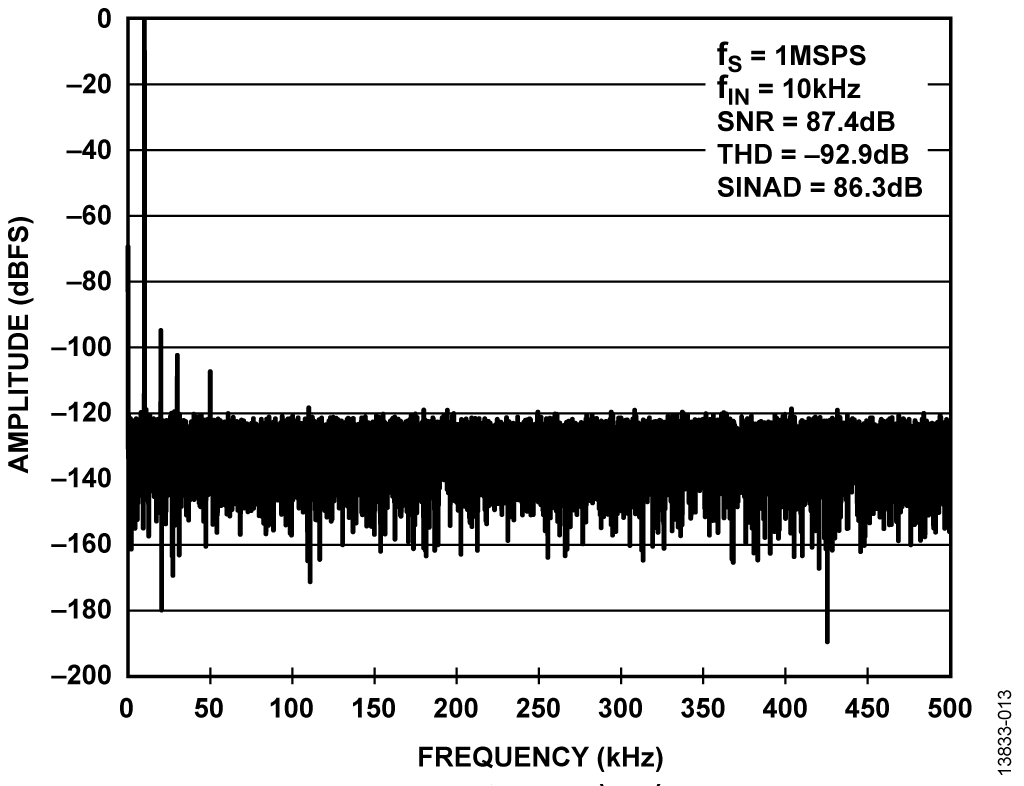Figure 13. FFT for 10 kHz, 2.5 V p-p Input for Gain = 3.2 on Single, Static Channel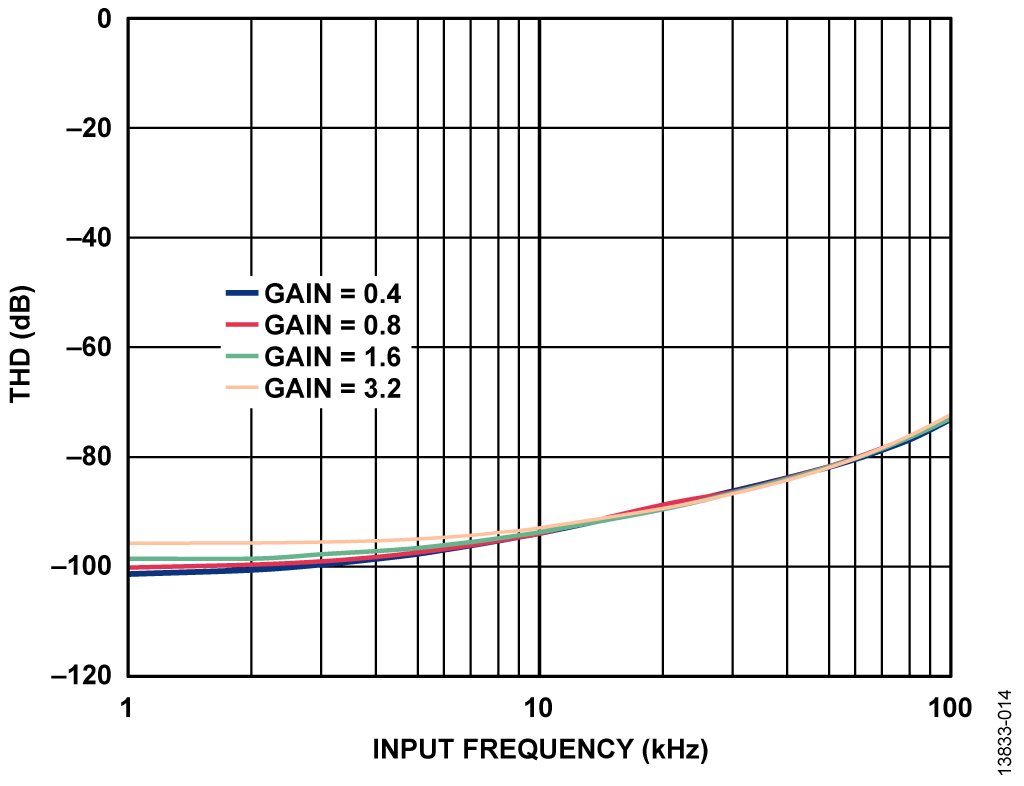Figure 14. THD Measured for Various Input Frequencies on a Single, Static Channel

System Performance with Channel Switching

Several tests were performed to evaluate the performance of the system when scanning multiple channels. Experiments using precision dc sources measured the error in output code with respect to sample rate (see Circuit Note CN-0269 for similar tests) and voltage step size between channels. AC performance was also measured for switching between two out-of-phase, full-scale inputs from a precision ac source (Audio Precision AP SYS 2712).

Figure 15 and Figure 16 show the test setup for dc and ac performance tests, respectively. The channel switching rate is the rate at which the ADG1207 switches from one channel to another, and is equivalent to the sample rate of the AD7982.Figure 15. Settling Time Evaluation Setup using DC CalibratorsFigure 16. Settling Time Evaluation Setup using AC Signal Generator

The dc tests involved varying the voltage step size between the two channels and the channel switching rate. The channel switching rate was varied from 50 kHz to 1 MHz in 50 kHz increments. The voltage step size was varied over different ranges for each of the gain configurations. A mean code result was measured for each channel for each voltage step size/channel switching rate by averaging 8,192 samples taken on each channel. A mean code result was also measured for each channel in the static case (no switching between channels). The mean code errors discussed below were found by taking the difference between the mean codes measured for the static case and for the switching channels.

Figure 17, Figure 18, Figure 19, and Figure 20 show the mean code error for various voltage step sizes at several switching rates in each of the four gain configurations. Figure 21, Figure 22, Figure 23, and Figure 24 show the mean code error for full-scale voltage steps at various switching rates in each of the four gain configurations.Figure 17. Mean Code Error vs. Voltage Step Size, Gain = 0.4Figure 18. Mean Code Error vs. Voltage Step Size, Gain = 0.8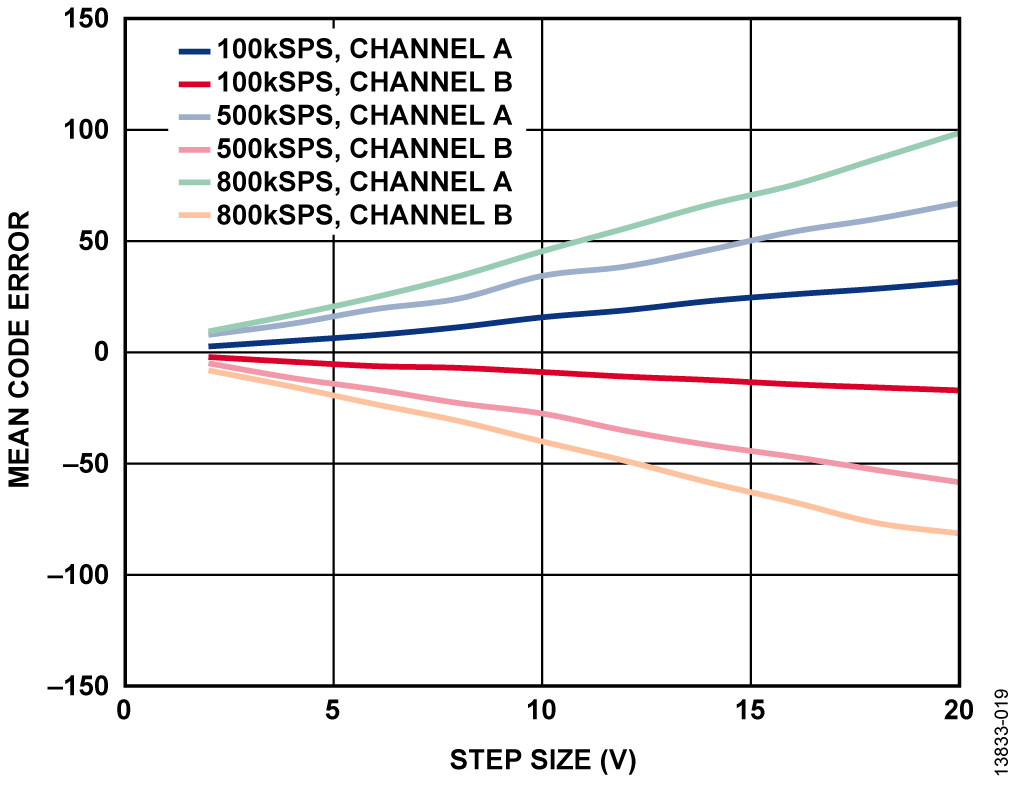Figure 19. Mean Code Error vs. Voltage Step Size, Gain = 1.6Figure 20. Mean Code Error vs. Voltage Step Size, Gain = 3.2Figure 21. Mean Code Error vs. Channel Switching Rate for Full-Scale Input Step, Gain = 0.4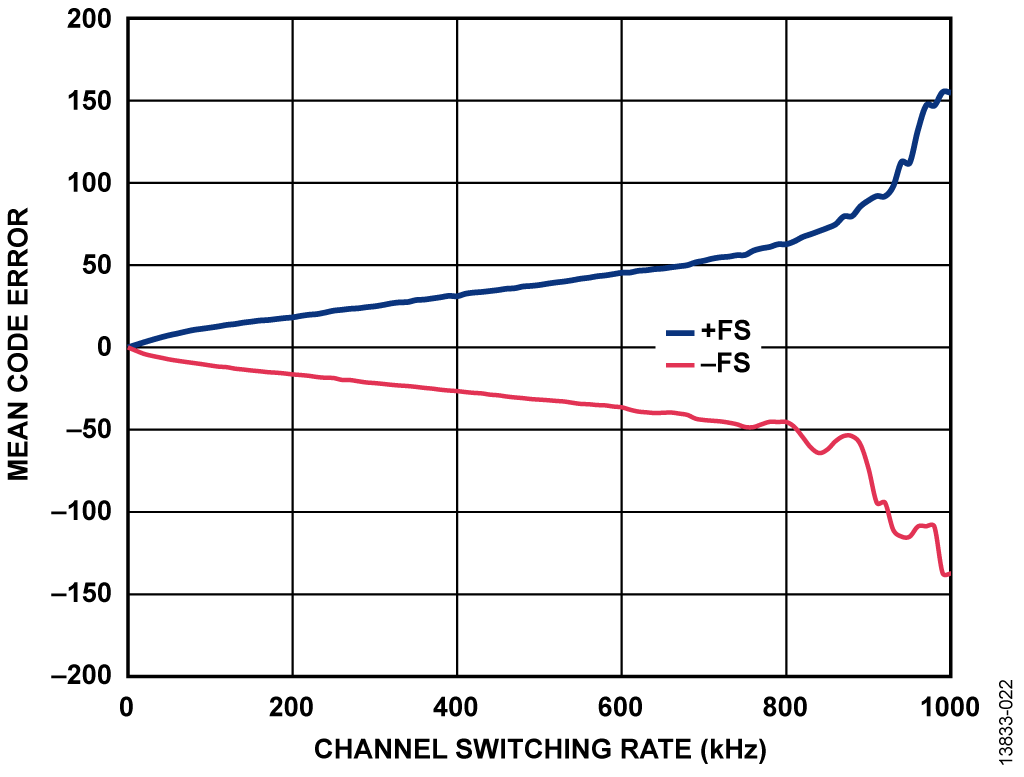Figure 22. Mean Code Error vs. Channel Switching Rate for Full-Scale Input Step, Gain = 0.8Figure 23. Mean Code Error vs. Channel Switching Rate for Full-Scale Input Step, Gain = 1.6Figure 24. Mean Code Error vs. Channel Switching Rate for Full-Scale Input Step, Gain = 3.2

The mean code error increases as the voltage step size and channel switching rate increase. This is due to the combined slew and settling time limitations of the components in the signal chain. Increasing the step size forces the system to settle larger changes in voltage and increasing the channel switching rate decreases the amount of time the system is given to settle these changes. At sufficiently high step sizes and switching rates, the mean code error becomes unpredictably large, as in the gain of 0.4 configuration (see Figure 17 and Figure 21). This is due to the slew rate limitations of the input buffer amplifiers in the AD8251 in-amp.

The performance of the system when using the ac source was evaluated by comparing its THD with respect to the channel switching rate. The AP SYS-2712 provided a full-scale sine wave input on one channel and an inverted version of the sine wave on another channel. THD was measured for various sample rates, ranging from 50 kSPS to 1 MSPS in 50 kSPS increments. Figure 25 shows the THD measured for each of the channels in each of the gain configurations.Figure 25. THD vs. ADG1207 Channel Switching Rate for Full-Scale 1 kHz Input

The THD performance of the system begins to degrade at roughly 750 kSPS (depending on the gain configuration). This is somewhat close to the expected maximum system sample rate calculated in the Settling Time Analysis section (770 kSPS).

Power Consumption Results

Figure 26 shows the power consumed by the circuit in Figure 1 as a function of the channel switching rate/system sample rate for each of the gain configurations. To maximize power demands for each of the components, two out of phase, full-scale sine wave inputs were applied to the odd and even channels of the system, which ensured that the outputs of the amplifier were constantly excited and slewing, and that the AD7982 was hitting a wide range of output codes.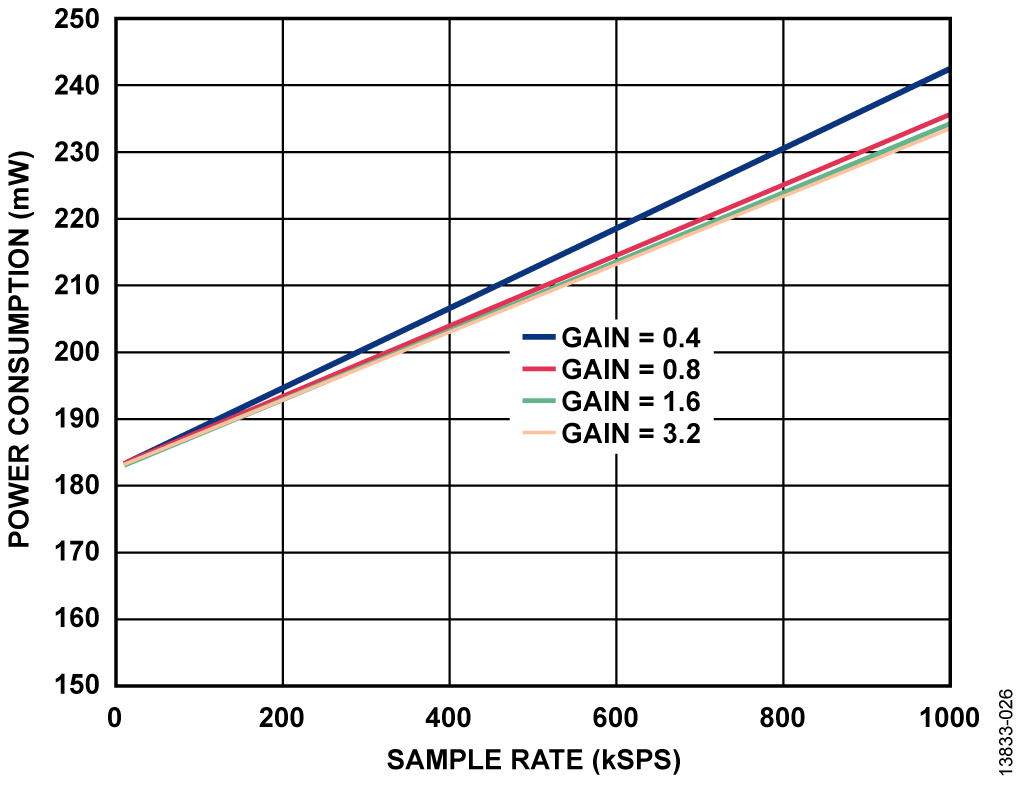Figure 26. Total System Power Consumption vs. System Sample Rate

The power consumption for the AD8251 and AD8475 increases with channel switching rate. This is because they spend relatively more time in the slewing phase when less time is given between switching channels. The power consumption of the AD7982 also scales with sample rate, as stated in its data sheet.

# Оценивание характеристик и тестирование схем

This circuit uses the EVAL-CN0345-SDZ circuit board and the EVAL-SDP-CB1Z SDP-B system demonstration platform controller board. The two boards have 120-pin mating connectors, allowing for the quick setup and evaluation of the performance of the circuit. The circuit board contains the circuit to be evaluated, as described in this note, and the SDP-B controller board is used with the CN-0345 Evaluation Software to capture the data from the circuit board.

Equipment​ Needed

The following equipment is needed:

• PC with a USB port and Windows® XP or Windows Vista® (32-bit), or Windows 7 (32-bit)
• EVAL-CN0345-SDZ circuit evaluation board
• EVAL-SDP-CB1Z SDP controller board
• 6 V to 12 V dc power supply or wall wart (9 V wall wart included with EVAL-CN0345-SDZ board)
• USB to Micro-USB cable
• Low distortion, low output impedance signal generator to provide ±10 V output
• Low noise, high precision dc supply to provide ±10 V output

Getting Star​ted

​Functional Block Diagram

See Figure 1 for the circuit block diagram and the EVAL-CN0345-SDZ-SCH.pdf file for the complete circuit schematic. This file is contained in the CN-0345 Design Support Package (www.analog.com/CN0345-DesignSupport). A functional block diagram of the test setup is shown in Figure 27.Figure 27. Test Setup Functional Block Diagram

Hardware Se​tup

Figure 28 shows the EVAL-CN0345-SDZ evaluation hardware. Information and details regarding the SDP-B board can be found in the SDP-B User Guide.

Connect the 120-pin connector on the circuit board to the CON A connector on the SDP-B controller board. Use nylon hardware to firmly secure the connection between the two boards, using the screw holes provided at the ends of the 120-pin connectors.

First, connect a 6 V to 12 V dc wall wart to P19 on the circuit board (or alternatively, connect a power supply to the P20 terminal block or the VIN test point). Then connect the SDP-B board to the PC via the USB to Micro-USB cable.

Tes​t

With the power supply or dc wall wart and USB cable connected, launch the evaluation software. Once USB communications are established, the SDP-B board can be used to send, receive, and capture data from the EVAL-CN0345-SDZ board and perform data analysis in the time and frequency domains.

Information and details regarding test setup and calibration, and how to use the evaluation software for data capture can be found in the CN-0345 Software User Guide (www.analog.com/CN0345-UserGuide).Figure 28. EVAL-CN0345-SDZ Evaluation Hardware